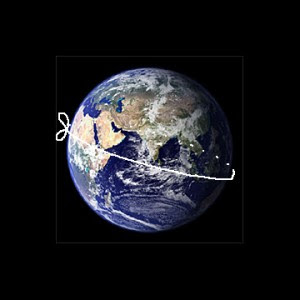A# rope-around-the-Earth puzzleImagine a rope that fits snugly all the way around the Earth like a ring on a person's finger. Now imagine the rope is made just one meter longer and lifted uniformly off the surface until it is once again taught. What will its height be above the surface? This puzzle, or one very like it, appeared in a students' book on Euclid written in 1702 by the English clergyman, mathematician, and natural philosopher William Whiston (1667–1752). The answer in the form just given is remarkable: about 16 centim.eters

How can this be? The answer comes simply from the formula for the circumference of a circle. If the extra radius of the rope is r and the Earth's radius is R,

then 2π(R + r) = 2πR + 100
so that r = 100/2π = ~15.9